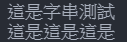#DAY 8
2

## 字串的大小寫處理

python在字串的大小寫處理中給了四個功能，分別是:

• upper() : 將所有字母改為大寫
• lower() : 將所有字母改為小寫
• capitalize() : 將字串中的第一個字母大寫，其餘小寫
• title() : 將每組單詞(空格區分)的第一個字母大寫，其餘小寫

``````a = 'a B cD eF'
print(a.upper())
print(a.lower())
print(a.capitalize())
print(a.title())
``````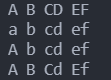``````a = 'abcdef'
print(a.isupper())
print(a.islower())
``````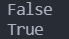## 字串分割

``````a = 'This is a banana'
print(a.split())
``````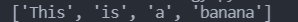split也可以指定想要切割的字元，以及要切割幾次:

``````a = 'This/is/a/banana'
print(a.split('/',2))
``````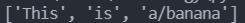## 字串格式化

``````a = 10
print(f"I have {a} bananas")
``````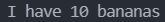## 使用者輸入

``````a = input("請輸入:")
print(a)
``````

input後面的括號裡面可以放顯示給使用者的訊息。這段程式碼的效果就會像下圖一樣，可以在終端機輸入文字並且回傳。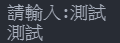## 字串的連接

``````a = "這是"
b = "字串測試"
print(a+b)
print(a*3)
``````